GFG App
Open AppBrowser
Continue

# Count distinct elements in every window of size k

Given an array of size N and an integer K, return the count of distinct numbers in all windows of size K.

Examples:

Input: arr[] = {1, 2, 1, 3, 4, 2, 3}, K = 4
Output: 3 4 4 3
Explanation: First window is {1, 2, 1, 3}, count of distinct numbers is 3
Second window is {2, 1, 3, 4} count of distinct numbers is 4
Third window is {1, 3, 4, 2} count of distinct numbers is 4
Fourth window is {3, 4, 2, 3} count of distinct numbers is 3

Input: arr[] = {1, 2, 4, 4}, K = 2
Output: 2 2 1
Explanation: First window is {1, 2}, count of distinct numbers is 2
First window is {2, 4}, count of distinct numbers is 2
First window is {4, 4}, count of distinct numbers is 1

This problem has appeared in Microsoft Interview Question.

## Naive Approach for finding the count of distinct numbers in all windows of size K:

Traverse the given array considering every window of size K in it and keeping a count on the distinct elements of the window

Follow the given steps to solve the problem:

• For every index, i from 0 to N – K, traverse the array from i to i + k using another loop. This is the window
• Traverse the window, from i to that index and check if the element is present or not
• If the element is not present in the prefix of the array, i.e no duplicate element is present from i to index-1, then increase the count, else ignore it
• Print the count

Below is the implementation of the above approach:

## C++

 `// C++ program to count distinct` `// elements in every window of size K`   `#include ` `using` `namespace` `std;`   `// Counts distinct elements in window of size K` `int` `countWindowDistinct(``int` `win[], ``int` `K)` `{` `    ``int` `dist_count = 0;`   `    ``// Traverse the window` `    ``for` `(``int` `i = 0; i < K; i++) {` `        ``// Check if element arr[i] exists in arr[0..i-1]` `        ``int` `j;` `        ``for` `(j = 0; j < i; j++)` `            ``if` `(win[i] == win[j])` `                ``break``;` `        ``if` `(j == i)` `            ``dist_count++;` `    ``}` `    ``return` `dist_count;` `}`   `// Counts distinct elements in all windows of size k` `void` `countDistinct(``int` `arr[], ``int` `N, ``int` `K)` `{` `    ``// Traverse through every window` `    ``for` `(``int` `i = 0; i <= N - K; i++)` `        ``cout << countWindowDistinct(arr + i, K) << endl;` `}`   `// Driver's code` `int` `main()` `{` `    ``int` `arr[] = {1, 2, 1, 3, 4, 2, 3}, K = 4;` `    ``int` `N = ``sizeof``(arr) / ``sizeof``(arr);`   `    ``// Function call` `    ``countDistinct(arr, N, K);` `    ``return` `0;` `}`

## Java

 `// Java program to count distinct elements in every` `// window of size K`   `import` `java.util.Arrays;`   `class` `Test {`   `    ``// Counts distinct elements in window of size K` `    ``static` `int` `countWindowDistinct(``int` `win[], ``int` `K)` `    ``{` `        ``int` `dist_count = ``0``;`   `        ``// Traverse the window` `        ``for` `(``int` `i = ``0``; i < K; i++) {` `            ``// Check if element arr[i] exists in arr[0..i-1]` `            ``int` `j;` `            ``for` `(j = ``0``; j < i; j++)` `                ``if` `(win[i] == win[j])` `                    ``break``;` `            ``if` `(j == i)` `                ``dist_count++;` `        ``}` `        ``return` `dist_count;` `    ``}`   `    ``// Counts distinct elements in all windows of size K` `    ``static` `void` `countDistinct(``int` `arr[], ``int` `N, ``int` `K)` `    ``{` `        ``// Traverse through every window` `        ``for` `(``int` `i = ``0``; i <= N - K; i++)` `            ``System.out.println(countWindowDistinct(` `                ``Arrays.copyOfRange(arr, i, arr.length), K));` `    ``}`   `    ``// Driver's code` `    ``public` `static` `void` `main(String args[])` `    ``{` `        ``int` `arr[] = {``1``, ``2``, ``1``, ``3``, ``4``, ``2``, ``3``}, K = ``4``;` `        `  `          ``// Function call` `        ``countDistinct(arr, arr.length, K);` `    ``}` `}`

## Python3

 `# Python3 program to count distinct` `# elements in every window of size K`   `import` `math as mt`   `# Counts distinct elements in window` `# of size K`     `def` `countWindowDistinct(win, K):`   `    ``dist_count ``=` `0`   `    ``# Traverse the window` `    ``for` `i ``in` `range``(K):`   `        ``# Check if element arr[i] exists` `        ``# in arr[0..i-1]` `        ``j ``=` `0` `        ``while` `j < i:` `            ``if` `(win[i] ``=``=` `win[j]):` `                ``break` `            ``else``:` `                ``j ``+``=` `1` `        ``if` `(j ``=``=` `i):` `            ``dist_count ``+``=` `1`   `    ``return` `dist_count`     `# Counts distinct elements in all` `# windows of size k` `def` `countDistinct(arr, N, K):`   `    ``# Traverse through every window` `    ``for` `i ``in` `range``(N ``-` `K ``+` `1``):` `        ``print``(countWindowDistinct(arr[i:K ``+` `i], K))`     `# Driver's Code` `if` `__name__``=``=``'__main__'``:` `  ``arr ``=` `[``1``, ``2``, ``1``, ``3``, ``4``, ``2``, ``3``]` `  ``K ``=` `4` `  ``N ``=` `len``(arr)` `  `  `  ``# Function call` `  ``countDistinct(arr, N, K)`   `# This code is contributed by` `# Mohit kumar 29`

## C#

 `// Simple C# program to count distinct` `// elements in every window of size K` `using` `System;` `using` `System.Collections.Generic;`   `class` `GFG {` `    ``// Counts distinct elements in` `    ``// window of size K` `    ``static` `int` `countWindowDistinct(``int``[] win, ``int` `K)` `    ``{` `        ``int` `dist_count = 0;`   `        ``// Traverse the window` `        ``for` `(``int` `i = 0; i < K; i++) {` `            ``// Check if element arr[i]` `            ``// exists in arr[0..i-1]` `            ``int` `j;` `            ``for` `(j = 0; j < i; j++)` `                ``if` `(win[i] == win[j])` `                    ``break``;` `            ``if` `(j == i)` `                ``dist_count++;` `        ``}` `        ``return` `dist_count;` `    ``}`   `    ``// Counts distinct elements in` `    ``// all windows of size k` `    ``static` `void` `countDistinct(``int``[] arr, ``int` `N, ``int` `K)` `    ``{` `        ``// Traverse through every window` `        ``for` `(``int` `i = 0; i <= N - K; i++) {` `            ``int``[] newArr = ``new` `int``[K];` `            ``Array.Copy(arr, i, newArr, 0, K);` `            ``Console.WriteLine(` `                ``countWindowDistinct(newArr, K));` `        ``}` `    ``}`   `    ``// Driver's Code` `    ``public` `static` `void` `Main(String[] args)` `    ``{` `        ``int``[] arr = {1, 2, 1, 3, 4, 2, 3};` `        ``int` `K = 4;` `        `  `          ``// Function call` `        ``countDistinct(arr, arr.Length, K);` `    ``}` `}`   `// This code is contributed by Princi Singh`

## Javascript

 `// Javascript program to count distinct elements in every` `// window of size k`   `// Counts distinct elements in window of size k` `    ``function` `countWindowDistinct(win, k)` `    ``{` `        ``let dist_count = 0;` ` `  `        ``// Traverse the window` `        ``for` `(let i = 0; i < k; i++) {` `            ``// Check if element arr[i] exists in arr[0..i-1]` `            ``let j;` `            ``for` `(j = 0; j < i; j++)` `                ``if` `(win[i] == win[j])` `                    ``break``;` `            ``if` `(j == i)` `                ``dist_count++;` `        ``}` `        ``return` `dist_count;` `    ``}` ` `  `    ``// Counts distinct elements in all windows of size k` `    ``function` `countDistinct(arr, N, K)` `    ``{` `        ``// Traverse through every window` `        ``for` `(let i = 0; i <= N - K; i++)` `            ``document.write(countWindowDistinct(arr.slice(i, arr.length), K) + ``"
"``);` `    ``}`     `// Driver program `   `      ``let arr = [1, 2, 1, 3, 4, 2, 3], K = 4;` ` `  `        ``countDistinct(arr, arr.length, K);` ` `  ` ``// This code is contributed by target_2.`

Output

```3
4
4
3```

Time complexity: O(N * K2)
Auxiliary Space: O(1)

## Count distinct numbers in all windows of size K using hashing:

So, there is an efficient solution using hashing, though hashing requires extra O(n) space but the time complexity will improve. The trick is to use the count of the previous window while sliding the window. To do this a hash map can be used that stores elements of the current window. The hash-map is also operated on by simultaneous addition and removal of an element while keeping track of distinct elements. The problem deals with finding the count of distinct elements in a window of length k, at any step while shifting the window and discarding all the computation done in the previous step, even though k – 1 elements are same from the previous adjacent window. For example, assume that elements from index i to i + k – 1 are stored in a Hash Map as an element-frequency pair. So, while updating the Hash Map in range i + 1 to i + k, reduce the frequency of the i-th element by 1 and increase the frequency of (i + k)-th element by 1.
Insertion and deletion from the HashMap takes constant time.

Follow the given steps to solve the problem:

• Create an empty hash map. Let the hash map be hm.
• Initialize the count of distinct elements as dist_count to 0.
• Traverse through the first window and insert elements of the first window to hm. The elements are used as key and their counts as the value in hm. Also, keep updating dist_count
• Print distinct count for the first window.
• Traverse through the remaining array (or other windows).
• Remove the first element of the previous window.
• If the removed element appeared only once, remove it from hm and decrease the distinct count, i.e. do “dist_count–“
• else (appeared multiple times in hm), then decrement its count in hm
• Add the current element (last element of the new window)
• If the added element is not present in hm, add it to hm and increase the distinct count, i.e. do “dist_count++”
• Else (the added element appeared multiple times), increment its count in hm

Below is the illustration of the above approach:

Consider the array arr[] = {1, 2, 1, 3, 4, 2, 3} and K = 4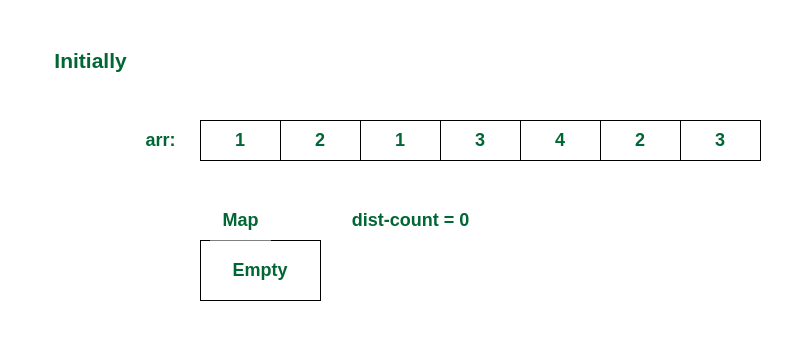Initial State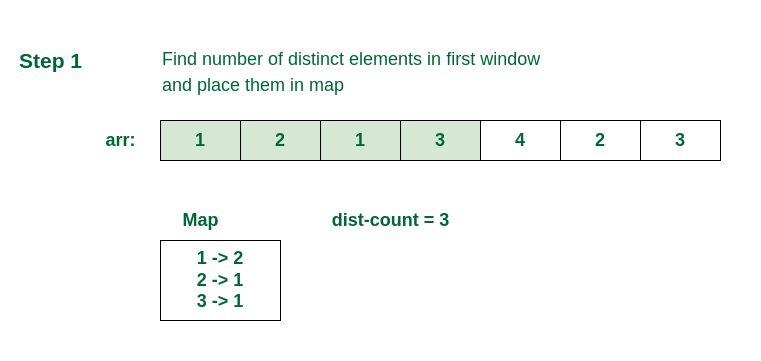Checking in the first window

In all the remaining steps, remove the first element of the previous window and add new element of current window.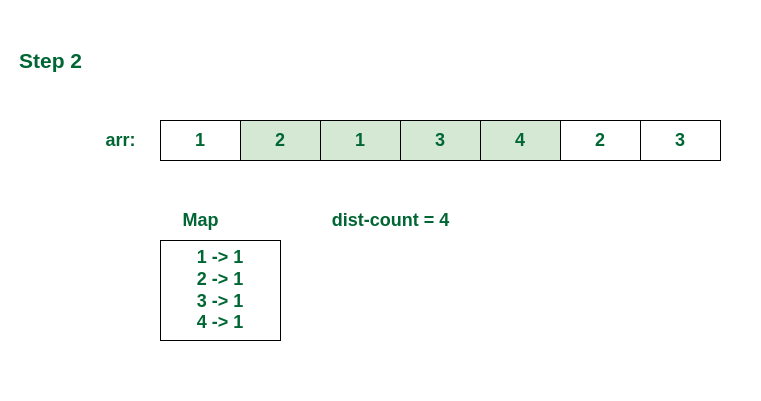Checking in the second window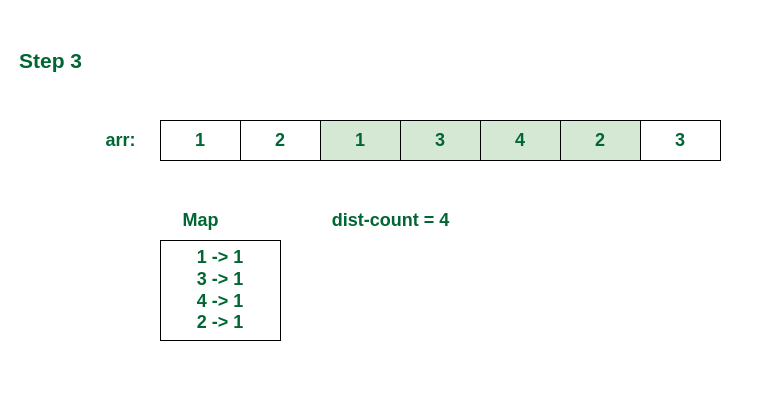Checking in the third window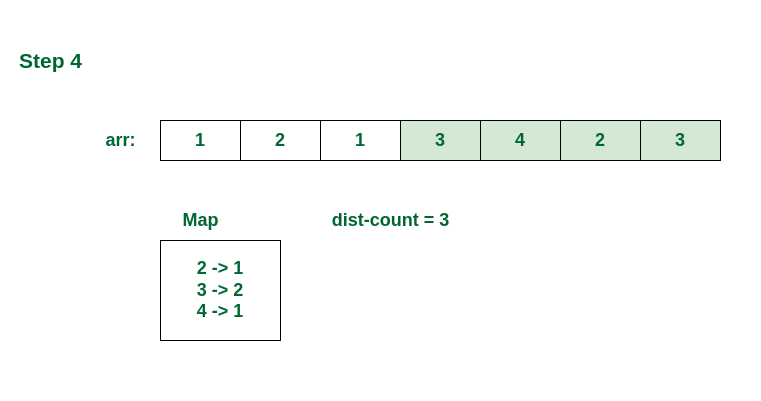Checking the last window

Below is the implementation of the above approach:

## C++

 `// C++ program for the above approach`   `#include ` `#include ` `using` `namespace` `std;`   `void` `countDistinct(``int` `arr[], ``int` `K, ``int` `N)` `{` `    ``// Creates an empty hashmap hm` `    ``unordered_map<``int``, ``int``> hm;`   `    ``// initialize distinct element count for current window` `    ``int` `dist_count = 0;`   `    ``// Traverse the first window and store count` `    ``// of every element in hash map` `    ``for` `(``int` `i = 0; i < K; i++) {` `        ``if` `(hm[arr[i]] == 0) {` `            ``dist_count++;` `        ``}` `      `  `        ``hm[arr[i]] += 1;` `    ``}`   `    ``// Print count of first window` `    ``cout << dist_count << endl;`   `    ``// Traverse through the remaining array` `    ``for` `(``int` `i = K; i < N; i++) {` `        ``// Remove first element of previous window` `        ``// If there was only one occurrence, then reduce distinct count.` `        ``if` `(hm[arr[i - K]] == 1) {` `            ``dist_count--;` `        ``}` `        ``// reduce count of the removed element` `        ``hm[arr[i - K]] -= 1;`   `        ``// Add new element of current window` `        ``// If this element appears first time,` `        ``// increment distinct element count`   `        ``if` `(hm[arr[i]] == 0) {` `            ``dist_count++;` `        ``}` `        ``hm[arr[i]] += 1;`   `        ``// Print count of current window` `        ``cout << dist_count << endl;` `    ``}` `}`   `// Driver's code` `int` `main()` `{` `    ``int` `arr[] = {1, 2, 1, 3, 4, 2, 3};` `    ``int` `N = ``sizeof``(arr) / ``sizeof``(arr);` `    ``int` `K = 4;` `  `  `      ``// Function call` `    ``countDistinct(arr, K, N);`   `    ``return` `0;` `}` `// This solution is contributed by Aditya Goel`

## Java

 `// Java program for the above approach`   `import` `java.util.HashMap;`   `class` `CountDistinctWindow {` `    ``static` `void` `countDistinct(``int` `arr[], ``int` `K)` `    ``{` `        ``// Creates an empty hashMap hM` `        ``HashMap hM` `            ``= ``new` `HashMap();`   `        ``// Traverse the first window and store count` `        ``// of every element in hash map` `        ``for` `(``int` `i = ``0``; i < K; i++)` `            ``hM.put(arr[i], hM.getOrDefault(arr[i], ``0``) + ``1``);`   `        ``// Print count of first window` `        ``System.out.println(hM.size());`   `        ``// Traverse through the remaining array` `        ``for` `(``int` `i = K; i < arr.length; i++) {`   `            ``// Remove first element of previous window` `            ``// If there was only one occurrence` `            ``if` `(hM.get(arr[i - K]) == ``1``) {` `                ``hM.remove(arr[i - K]);` `            ``}`   `            ``else` `// reduce count of the removed element` `                ``hM.put(arr[i - K], hM.get(arr[i - K]) - ``1``);`   `            ``// Add new element of current window` `            ``// If this element appears first time,` `            ``// set its count as 1,` `            ``hM.put(arr[i], hM.getOrDefault(arr[i], ``0``) + ``1``);`   `            ``// Print count of current window` `            ``System.out.println(hM.size());` `        ``}` `    ``}`   `    ``// Driver's code` `    ``public` `static` `void` `main(String arg[])` `    ``{` `        ``int` `arr[] = { ``1``, ``2``, ``1``, ``3``, ``4``, ``2``, ``3` `};` `        ``int` `K = ``4``;`   `        ``// Function call` `        ``countDistinct(arr, K);` `    ``}` `}`

## Python3

 `# An efficient Python program to` `# count distinct elements in` `# every window of size K` `from` `collections ``import` `defaultdict`     `def` `countDistinct(arr, K, N):`   `    ``# Creates an empty hashmap hm` `    ``mp ``=` `defaultdict(``lambda``: ``0``)`   `    ``# initialize distinct element` `    ``# count for current window` `    ``dist_count ``=` `0`   `    ``# Traverse the first window and store count` `    ``# of every element in hash map` `    ``for` `i ``in` `range``(K):` `        ``if` `mp[arr[i]] ``=``=` `0``:` `            ``dist_count ``+``=` `1` `        ``mp[arr[i]] ``+``=` `1`   `    ``# Print count of first window` `    ``print``(dist_count)`   `    ``# Traverse through the remaining array` `    ``for` `i ``in` `range``(K, N):`   `        ``# Remove first element of previous window` `        ``# If there was only one occurrence,` `        ``# then reduce distinct count.` `        ``if` `mp[arr[i ``-` `K]] ``=``=` `1``:` `            ``dist_count ``-``=` `1` `        ``mp[arr[i ``-` `K]] ``-``=` `1`   `    ``# Add new element of current window` `    ``# If this element appears first time,` `    ``# increment distinct element count` `        ``if` `mp[arr[i]] ``=``=` `0``:` `            ``dist_count ``+``=` `1` `        ``mp[arr[i]] ``+``=` `1`   `        ``# Print count of current window` `        ``print``(dist_count)`   `# Driver's code` `if` `__name__``=``=``'__main__'``:` `  ``arr ``=` `[``1``, ``2``, ``1``, ``3``, ``4``, ``2``, ``3``]` `  ``N ``=` `len``(arr)` `  ``K ``=` `4`   `  ``# Function call` `  ``countDistinct(arr, K, N)`   `# This code is contributed by Shrikant13`

## C#

 `// C# program for the above approach`   `using` `System;` `using` `System.Collections.Generic;`   `public` `class` `CountDistinctWindow {` `    ``static` `void` `countDistinct(``int``[] arr, ``int` `K)` `    ``{` `        ``// Creates an empty hashMap hM` `        ``Dictionary<``int``, ``int``> hM` `            ``= ``new` `Dictionary<``int``, ``int``>();`   `        ``// initialize distinct element count for` `        ``// current window` `        ``int` `dist_count = 0;`   `        ``// Traverse the first window and store count` `        ``// of every element in hash map` `        ``for` `(``int` `i = 0; i < K; i++) {` `            ``if` `(!hM.ContainsKey(arr[i])) {` `                ``hM.Add(arr[i], 1);` `                ``dist_count++;` `            ``}` `            ``else` `{` `                ``int` `count = hM[arr[i]];` `                ``hM.Remove(arr[i]);` `                ``hM.Add(arr[i], count + 1);` `            ``}` `        ``}`   `        ``// Print count of first window` `        ``Console.WriteLine(dist_count);`   `        ``// Traverse through the remaining array` `        ``for` `(``int` `i = K; i < arr.Length; i++) {`   `            ``// Remove first element of previous window` `            ``// If there was only one occurrence, then` `            ``// reduce distinct count.` `            ``if` `(hM[arr[i - K]] == 1) {` `                ``hM.Remove(arr[i - K]);` `                ``dist_count--;` `            ``}` `            ``else` `// reduce count of the removed element` `            ``{` `                ``int` `count = hM[arr[i - K]];` `                ``hM.Remove(arr[i - K]);` `                ``hM.Add(arr[i - K], count - 1);` `            ``}`   `            ``// Add new element of current window` `            ``// If this element appears first time,` `            ``// increment distinct element count` `            ``if` `(!hM.ContainsKey(arr[i])) {` `                ``hM.Add(arr[i], 1);` `                ``dist_count++;` `            ``}` `            ``else` `// Increment distinct element count` `            ``{` `                ``int` `count = hM[arr[i]];` `                ``hM.Remove(arr[i]);` `                ``hM.Add(arr[i], count + 1);` `            ``}`   `            ``// Print count of current window` `            ``Console.WriteLine(dist_count);` `        ``}` `    ``}`   `    ``// Driver's code` `    ``public` `static` `void` `Main(String[] arg)` `    ``{` `        ``int``[] arr = {1, 2, 1, 3, 4, 2, 3};` `        ``int` `K = 4;` `      `  `          ``// Function call` `        ``countDistinct(arr, K);` `    ``}` `}`   `// This code contributed by Rajput-Ji`

## Javascript

 `// Javascript program to count distinct elements in` `// every window of size k` `    `  `   ``function` `countDistinct(arr, k)` `    ``{` `        ``// Creates an empty hashMap hM` `        ``let hM = ``new` `Map();`   `        ``// Traverse the first window and store count` `        ``// of every element in hash map` `        ``for` `(let i = 0; i < k; i++)` `        ``{` `            ``if``(hM.has(arr[i]))` `            ``hM.set(arr[i], hM.get(arr[i])+1)` `            ``else` `            ``hM.set(arr[i], 1);` `        ``}`   `        ``// Print count of first window` `        ``document.write(hM.size + ``"
"``);`   `        ``// Traverse through the remaining array` `        ``for` `(let i = k; i < arr.length; i++) {`   `            ``// Remove first element of previous window` `            ``// If there was only one occurrence` `            ``if` `(hM.get(arr[i - k]) == 1) {` `                ``hM.``delete``(arr[i - k]);` `            ``}`   `            ``else` `// reduce count of the removed element` `                ``hM.set(arr[i - k],  hM.get(arr[i - k]) - 1);            `   `            ``// Add new element of current window` `            ``// If this element appears first time,` `            ``// set its count as 1,` `            ``if``(hM.has(arr[i]))` `            ``hM.set(arr[i], hM.get(arr[i])+1)` `            ``else` `            ``hM.set(arr[i], 1);`   `            ``// Print count of current window` `            ``document.write(hM.size + ``"
"``);` `        ``}` `    ``}`     `// Driver code` `    ``let arr = [1, 2, 1, 3, 4, 2, 3];` `    ``let size = arr.length;` `    ``let k = 4;` `    ``countDistinct(arr, k, size);` `    `  `    ``// This code is contributed by splevel62.`

Output

```3
4
4
3```

Time complexity: O(N), A single traversal of the array is required.
Auxiliary Space: O(N), Since the hashmap requires linear space.